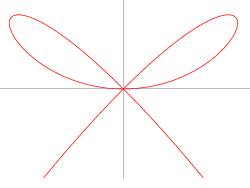# It's so cute!

Calculus Level 4The graph above satisfy the equation $x^4 + y^3 = x^2 y$.

The area enclosed by the 2 cute adorable little fine loops is equals to $\frac {a}{b}$ for coprime positive integers $a$ and $b$. What is the value of $a+b$?

×